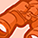# QlikView App Dev

Discussion Board for collaboration related to QlikView App Development.

Announcements
Join this live chat April 6, 10AM EST - QlikView to Qlik Sense REGISTER
cancel
Showing results for
Search instead for
Did you mean:Creator

## Problem with the number of data when you select a store and when you bring all the data

Hello,

I have the following problem.

I bring the 0 stock in a straight chart with the following expression.

sum({<ITEM_ID = {"=sum(STOC) = 0"}>} STOC)

by store and item. If i export all the data for all stores in an excel for example in the store 21 i have 1104 items that have 0 stock.

If i select from the stores only the 21 and export the data i have 2122 items that have 0 stock that is the correct.

Have anyone an idea why is this happening ?

Is the problem in the expression ?

Thank you in advance.

I

Labels (1)
• ### QlikView

1 Solution

Accepted SolutionsCreator
Author

I found the solution.

I created a flag in the reload script that i of th

LOAD

STORE,

ITEM_ID,

STOC,

if(STOC=0,'Zero','Value') as FLAG

and in the chart i wrote the following expression

sum({<FLAG={'Zero'}>}STOC)

and i have the same number of rows either i select the store or i don't any of store.

Thank you for your time.

11 RepliesPartner

Hello Geogou,

If you want to know how many items have 0 STOC per store, here is how you can do this:

``Count({<STOC ={0}>} ITEM_ID)``

If you want to know how much is the stock overall for items which have 0 stock in some of your shops, pm me and I can help you build the expression.

If working at all, this expression: =sum(STOC) = 0 would give you (-1) if true or (0) if false.

So your expression most likely works like this:

sum({<ITEM_ID = {"-1"}>} STOC)

or like this

sum({<ITEM_ID = {"0"}>} STOC)

I hope that helps!

Kind regards,

S.T.MVP

So, you are summing STOC where STOC = 0? I think Sum of many 0's will still be 0, isn't it?Creator
Author

Hello,

neither of the expressions do not work.Creator
Author

I want to show all the items for the store or all the stores that have stock 0MVP

Show it in which object? What are your dimensions (if it is in a chart) and what are your expressions (other than the one above)?Creator
Author

The dimensions are the store and the item and the expression is the following

sum({<ITEM_ID = {"=sum(STOC) = 0"}>} STOC)

and the problem is that when i choice a store a have the correct number of rows

when i don't choice and i show all the stores and items i have less items per store.

I don't know how that is happening.MVP

Can you try this

``````Sum(Aggr(
If(Sum(STOC) = 0, STOC)
, ITEM_ID, STORE))``````Creator
Author

Does not work correctly. It brings over of 18.000 rows in the store 21.MVP

How about this?

``````Sum(Aggr(
If(Sum(STOC) = 0, Sum(STOC))
, ITEM_ID, STORE))``````